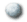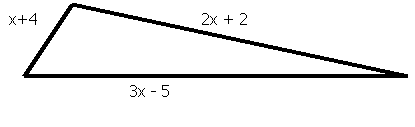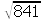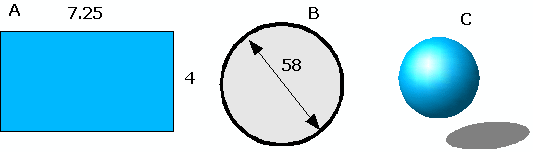MS 095 PreAlgebra Spring 2005 MXName:

1. __________ Calculate: - 29

2. __________ The expression (3+55)÷2 = 4 contains parenthesis. Are the parenthesis necessary to obtain the answer of 4?

3. __________ The expression (3+55)÷2 = 4 contains parenthesis. Are the parenthesis necessary to obtain the answer of 4?

4. __________ Are all even numbers composite numbers?

5. __________ Name a number that is a perfect square and can also be a rectangle number

6. ______________________________ Write an expression for the perimeter of the triangle in simplest form.7. __________ Simplify:8. __________ Identify the coefficient and degree of the monomial -29x

9. ____________________ Is 29x + 7 = 297 an expression or equation?

10. __________ Solve the problem: Afua opened a new checking account with a \$387 deposit. The bank charged her \$9 to print her checks. She also wrote three checks, one a \$60 check to buy groceries, a second \$8 check to pay her monthly phone bill, and a \$20 check for a phone card for her cell phone. What is the balance in her account.

11. __________ What is 29 ÷ 0 equal to?

12. __________ What is 0 ÷ 0 equal to?

13. ____________________ Calculate 293

14. __________ Solve for ψ: 5 * ψ - 8 = 137

15. Write the following in math notation and solve: 8 pompoms minus 7 pompoms is equal to...

16. __________ Calculate: (x + 5x² – 9 ) + (x² – 24x – 20)

17. __________ Calculate: (x + 5x² – 9 ) – (x² – 24x – 20)

18. __________ Calculate: 29x(y + 29 )

19. __________ Calculate: (x + 29 )(x – 29)

20. __________ If I run at a pace of 6.5 minutes per kilometer with a heart rate of 150 beats per minute, how many beats of my heart occur over a distance of one kilometer?

21. __________ Determine:Chuuk High School

2.47

NICHS Woleai High School

4.02

Outer Island High School Ulithi

3.23

Yap High School

4.55

22. Students at four high schools were given ten arithmetic problems to solve. The average number correct is shown in the table.

23. __________ Calculate the median for the four high schools.

24. __________ Calculate the mean for the four high schools.

25. __________ Toughie: The test given was multiple choice with five answers for each question. If one randomly guessed on all ten questions, how many would one be expected to get correct by random guessing?

26. __________ What is your body fat percentage?

27. __________ Are you at an essential, athletic, physically fit, acceptable, overfat, or obese level of body fat?

28. __________ Find the prime factorization of 14007.

29. __________ Find the Greatest Common Factor of 2520 and 2700.

30. __________ Find the perimeter of the rectangle A with edges of 7.25 cm and 4 cm as seen below.

31. __________ Find the area of the rectangle A with edges of 7.25 cm and 4 cm as seen below.

32. __________ Find the area of the circle B seen below. The circle has a diameter of 58 cm.

33. __________ Find the volume of the sphere C seen below. The sphere has a radius of 2.9 cm.